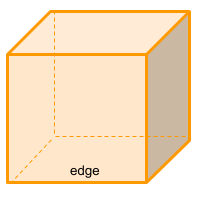Home » Polyhedra » Platonic Solids » Cube

# Volume of a CubeVolume =
edge³

## Volume calculator for a cube

Enter the edge of the cube to get the volume automatically.

## Description, how many faces, edges and vertices are there in a cube

The cube or hexahedron (hexa = six and hedron = faces) is a polyhedron because all of its faces are flat. Has 6 faces, 12 edges and 8 vertices. Each face has 4 edges, the opposite faces are parallel, the intersecting faces form 90 degree angles and at each vertex 3 edges meet.
The cube is also considered a prism, but with all of its faces congruent or with all its lengths equal.

## Examples of a cube

We can find many objects that look like a cube, examples of cube shaped objects are: a dice, Rubik's cube, a box, or even a sugar cube. Can you think of any other examples? leave a comment in the comment section at the bottom of the page.

## Formula for the volume of a cube

Calculate the volume of a cube is very simple, you only have to know the value of one edge and power it to 3, which is the same as multiplying edge x edge x edge. You can also use the online calculator to get the cube volume automatically.

Volume =
edge³

Share